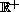Electron. J. Diff. Eqns., Vol. 2000(2000), No. 24, pp. 1-16.

### Periodic and almost periodic solutions for multi-valued differential equations in Banach spaces E. Hanebaly & B. Marzouki

Abstract:
It is known that for-periodic differential equations of monotonous type, in uniformly convex Banach spaces, the existence of a bounded solution onis equivalent to the existence of an-periodic solution (see Haraux  and Hanebaly [7, 10]). It is also known that if the Banach space is strictly convex and the equation is almost periodic and of monotonous type, then the existence of a continuous solution with a precompact range is equivalent to the existence of an almost periodic solution (see Hanebaly ). In this note we want to generalize the results above for multi-valued differential equations.

Submitted July 16, 1999. Published March 30, 2000.
Math Subject Classifications: 34A60, 34C25, 34C27, 47H10.
Key Words: Multi-valued differential equation, Hyper-accretive, Almost periodicity, Banach space.

Show me the PDF file (169K), TEX file, and other files for this article.

E. Hanebaly
Universite Mohammed V Faculte des Sciences
Departement de Mathematiques, Rabat, Maroc.
e-mail: hanebaly@fsr.ac.ma

Brahim Marzouki
Universite Mohammed I Faculte des Sciences
Departement de Mathematiques, Oujda, Maroc.
e-mail: marzouki@sciences.univ-oujda.ac.ma